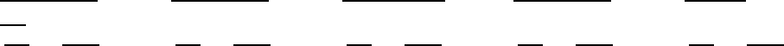Textbook Notes (290,000)
CA (170,000)
UTSG (10,000)
Chapter

# RSM220H1 Chapter Notes -Write-Off, Inventory Turnover, Debits And Credits

Department
Rotman Commerce
Course Code
RSM220H1
Professor
Xin Baohua

This preview shows pages 1-3. to view the full 30 pages of the document.
CHAPTER 9
ISSUES
MULTIPLE CHOICE—Conceptual
d 1. Knowledge of lower of cost or market valuations.
d 2. Appropriate use of LCM valuation.
c 3. Definition of "market" under LCM.
b 4. Definition of "ceiling."
a 5. Definition of "designated market value."
c 6. Application of lower of cost or market valuation.
d 7. Effect of inventory write-down.
b 8. Net realizable value under LCM.
d 9. Definition of "net realizable value."
a 10. Valuation of inventory at net realizable value.
d 11. Appropriate use of net realizable value.
a 12. Material purchase commitments.
a 13. Loss recognition on purchase commitments.
d 14. Appropriate use of the gross profit method.
b 15. Appropriate use of the gross profit method.
d 16. Advantage of retail inventory method.
c 17. Conventional retail inventory method.
a 18. Assumptions of the retail inventory method.
d 19. Appropriate use of the retail inventory method.
b 20. Markdowns and the conventional retail method.
a 21. Markups and the conventional retail method.
b *22. Knowledge of the cost ratio for retail inventory methods.
c 23. Inventory turnover ratio.
c *24. Dollar-value LIFO retail method.
MULTIPLE CHOICE—Computational
a 25. Value inventory at LCM.
c 26. Relative sales method of inventory valuation.
c 27. Entry for purchase commitment loss.
d 28. Calculate cost of goods sold given a markup on cost.
d 29. Calculate merchandise purchases given a markup on cost.
a 30. Calculate total sales from cost information.
a 31. Markup on cost equivalent to a markup on selling price.
b 32. Estimate ending inventory using gross profit method.
c 33. Calculate ending inventory using gross profit method
*This topic is dealt with in an Appendix to the chapter.
MULTIPLE CHOICE—Computational (cont.)
. b 34. Calculate ending inventory using gross profit method.

Only pages 1-3 are available for preview. Some parts have been intentionally blurred.

a 35. Estimate cost of inventory destroyed by fire.
a 36. Determine items to be included in inventory.
b 37. Calculate cost of retail ratio to approximate LCM.
b 38. Calculate ending inventory at retail.
a 39. Calculate cost to retail ratio approximating LCM.
b 40. Calculate cost of inventory lost using retail method.
b *41. Calculate ending inventory at cost using LIFO retail.
c *42. Determine cost to retail ratio using LIFO retail.
b 43. Calculate inventory turnover ratio.
d 44. Determine cost to retail ratio to approximate LCM.
c *45. Determine cost to retail ratio using LIFO cost.
d 46. Calculate ending inventory at retail.
a 47. Calculate ending inventory using conventional retail.
a *48. Calculate ending inventory cost using dollar-value LIFO.
b *49. Calculate cost of ending inventory using LIFO retail.
a *50. Calculate ending inventory cost using dollar-value LIFO.
d 51. Recognizing a loss due to LCM.
b 52. Appropriate use of replacement costs in LCM.
b 53. Identification of the designated market value.
a 54. Estimate cost of inventory lost by theft.
a 55. Determine cost of ending inventory using retail method.
d 56. Determine cost of ending inventory using retail method.
a *57. Calculate ending inventory using LIFO retail.
EXERCISES
Item Description
E9-58 Lower of cost or market.
E9-59 Lower of cost or market.
E9-60 Lower of cost or market.
E9-61 Lower of cost or market.
E9-62 Lower of cost or market.
E9-63 Relative sales value method.
E9-64 Gross profit method.
E9-65 Gross profit method.
E9-66 Gross profit method.
E9-67 Comparison of inventory methods.

Only pages 1-3 are available for preview. Some parts have been intentionally blurred.PROBLEMS
Item Description
P9-68 Gross profit method.
P9-69 Retail inventory method.
*P9-70 Retail inventory method.
*P9-71 LIFO retail inventory method, fluctuating prices.
*P9-72 LIFO retail inventory method, stable prices.
*P9-73 Dollar-value LIFO retail method.
*P9-74 Retail LIFO.
CHAPTER LEARNING OBJECTIVES
1. Explain and apply the lower of cost or market rule.
2. Identify when inventories are valued at net realizable value.
3. Explain when the relative sales value method is used to value inventories.
4. Explain accounting issues related to purchase commitments.
5. Determine ending inventory by applying the gross profit method.
6. Determine ending inventory by applying the retail method.
7. Explain how inventory is reported and analyzed.
*8. Determine ending inventory by applying the LIFO retail methods.
SUMMARY OF LEARNING OBJECTIVES BY QUESTIONS
L.O. 1 L.O. 2 L.O. 3 L.O. 4 L.O.
5
No. Type No. Type No. Type No. Type No. Type
1. MC 8. MC 26. MC 12. MC 14. MC
2. MC 9. MC 63. E 13. MC 15. MC
3. MC 10. MC 27. MC 32. MC
4. MC 11. MC 51. MC 33. MC
5. MC 34. MC
6. MC 35. MC
7. MC 36. MC
25. MC 54. MC
39. MC 64. E
52. MC 65. E
53. MC 66. E
56. MC 68. P
58. E
59. E
60. E
61. E
62. E
67. E